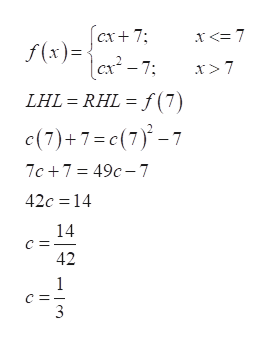# Find the value of the constant cc that makes the following function continuous on (−∞,∞)(−∞,∞). f(x)={cx+7 if x∈(−∞,7], cx^2−7 if x∈(7,∞) I tried to format this as best I could, but it is supposed to be a piecewise function.

Question
9 views

Find the value of the constant cc that makes the following function continuous on (−∞,∞)(−∞,∞).

f(x)={cx+7 if x∈(−∞,7], cx^2−7 if x∈(7,∞)

I tried to format this as best I could, but it is supposed to be a piecewise function.

check_circle

Step 1

We have been given a piecewise function and we need to determine the value of ‘c’ at which the given function is continuous on all real numbers.

Step 2

Since both the pieces of the given piecewise function are polynomials, therefore, we only need to check continuity at the point where the two pieces split, that is, x=7.

...help_outlineImage Transcriptionclosecx+ 7 f(x)= 7 x <= 7 x > 7 RHL = f(7) LHL c(7)+7= c(7)-7 7c 7 49c-7 42c 14 14 42 1 3 fullscreen

### Want to see the full answer?

See Solution

#### Want to see this answer and more?

Solutions are written by subject experts who are available 24/7. Questions are typically answered within 1 hour.*

See Solution
*Response times may vary by subject and question.
Tagged in

### Continuity